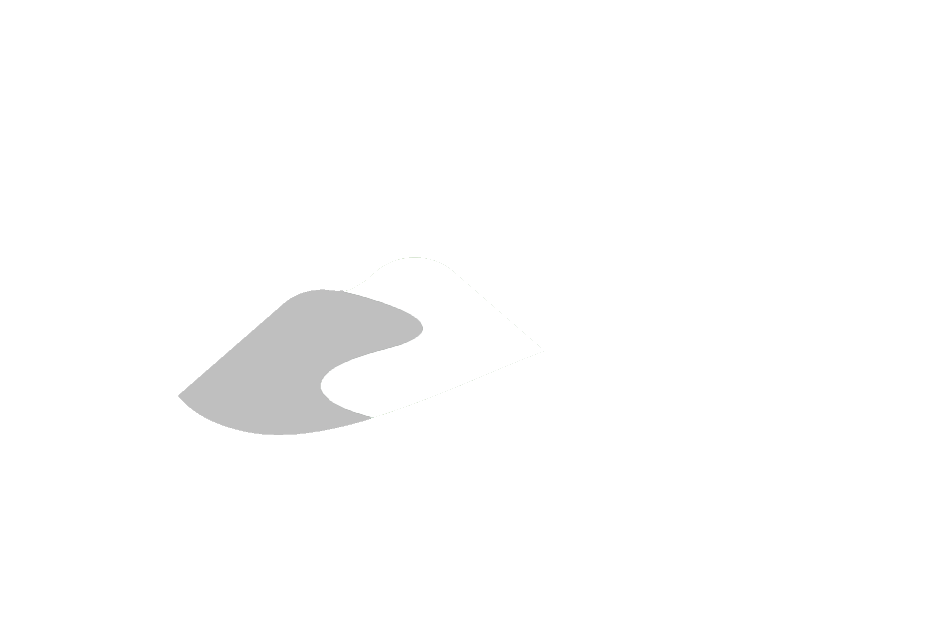View on GitHub

# General Information

## Oak Ridges Moraine Groundwater Program

Hydrologic Modelling using Artificial Neural Networks

# Artificial Neural Network (ANN)

A single hidden-layer with 3 (hidden) neurons ANN is built to replicate a runoff hydrograph. The inputs are lagged by three days and a neural network is “partially recurrent”, in that the prediction made in a previous $n$ timesteps is added as input to the following prediction:

$\hat{Q}_t=E \left\{ Q_t | Q_{t-1}, Q_{t-2}, \dots , Q_{t-n}, R_t, R_{t-1}, \dots , R_{t-n}, f(\cdot) \right\}$

In addition to the recurrency, $n$-day antecedent precipitation is added to the input along a sinusoidal function $(f(\cdot))$ ranging from 0 to 1 at the winter and summer solstices, respectively. In all, there are $2(n+1)$ inputs, $n$ hidden layer nodes and 1 output: Runoff.

Here $n=3$.

GitHub